SSC  >  Quantitative Aptitude - Test 9

# Quantitative Aptitude - Test 9

Test Description

## 25 Questions MCQ Test SSC CGL Tier 1 Mock Test Series | Quantitative Aptitude - Test 9

Quantitative Aptitude - Test 9 for SSC 2022 is part of SSC CGL Tier 1 Mock Test Series preparation. The Quantitative Aptitude - Test 9 questions and answers have been prepared according to the SSC exam syllabus.The Quantitative Aptitude - Test 9 MCQs are made for SSC 2022 Exam. Find important definitions, questions, notes, meanings, examples, exercises, MCQs and online tests for Quantitative Aptitude - Test 9 below.
Solutions of Quantitative Aptitude - Test 9 questions in English are available as part of our SSC CGL Tier 1 Mock Test Series for SSC & Quantitative Aptitude - Test 9 solutions in Hindi for SSC CGL Tier 1 Mock Test Series course. Download more important topics, notes, lectures and mock test series for SSC Exam by signing up for free. Attempt Quantitative Aptitude - Test 9 | 25 questions in 15 minutes | Mock test for SSC preparation | Free important questions MCQ to study SSC CGL Tier 1 Mock Test Series for SSC Exam | Download free PDF with solutions
 1 Crore+ students have signed up on EduRev. Have you?
Quantitative Aptitude - Test 9 - Question 1

### Find the value of K for which the points A (4, 3), B(5, 4) and C(K, 6) are collinear.

Detailed Solution for Quantitative Aptitude - Test 9 - Question 1

Three points A(x1, y1), B(x2, y2) and C(x3, y3) are collinear, if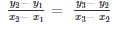As, A (4, 3), B (5, 4) and C (K, 6) are collinear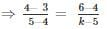⇒ k - 5 = 2
⇒ k = 7

Quantitative Aptitude - Test 9 - Question 2

### Two circles touch each other externally at P. QR is a common tangent to the circles touching them at Q and R. The measure of ∠QPR is

Detailed Solution for Quantitative Aptitude - Test 9 - Question 2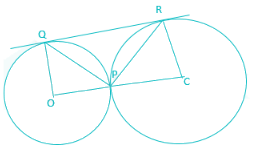In triangle OPQ,
OP = OQ (radius of circle)
∴ ∠OQP = ∠OPQ
Let, ∠OQP = ∠OPQ = x
In triangle PCR,
CP = CR (radius of circle)
∴ ∠CPR = ∠CRP
Let, ∠CPR = ∠CRP = y
In given figure,
∠OPQ + ∠QPR + ∠RPC = 180°
⇒ x + ∠QPR + y = 180°
⇒ ∠QPR = 180° - x – y ……….eq (1)
As, we know that, radius and tangent to the circle are perpendicular to each other,
Therefore,
∠OQR and ∠CRQ are right angles
∴ ∠PQO + ∠PQR = 90°
⇒ ∠PQR = 90° - x° ………….eq (2)
∴ ∠ CRP + ∠ PRQ = 90°
⇒ ∠PRQ = 90° - y° ………..eq(3)
In triangle QPR
∠QPR + ∠PQR + ∠PRQ = 180°
⇒ 180° - x – y + 90° - x° +90° - y° = 180°
⇒ 360° - 2(x + y) = 180°
⇒ 2(x + y) = 180°
⇒ (x + y) = 90°
∠QPR = 180° - x – y
= 180° - 90°
= 90°

Quantitative Aptitude - Test 9 - Question 3

### The difference between interior and exterior angle of a regular polygon is 100°. Find the number of sides of polygon.

Detailed Solution for Quantitative Aptitude - Test 9 - Question 3

Let out of exterior and interior angle one of the angle be x
∴ Other angle = x + 100.
Now as we know that interior angle + exterior angle = 180°
∴ x + x + 100 = 180°
⇒ 2x + 100 = 180°
⇒ 2x = 80°
⇒ x = 40°
∴ other angle = 140°
Now, since exterior angle of a regular polygon can not exceed 120°
∴ Interior angle = 140° and, Exterior angle = 40°
Now no. of sides of a regular polygon = 360 / exterior angle
∴ No. of sides = 360 / 40 = 9

Quantitative Aptitude - Test 9 - Question 4

If x + y + z = 0, the value of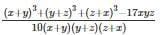Detailed Solution for Quantitative Aptitude - Test 9 - Question 4

We have x + y + z = 0 …(1)
We have to find the value of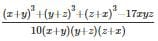Substituting values of x + y, y + z, z + x from (1)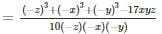We also know that
x3 + y3 + z3 - 3xyz = (x + y + z)(x2 + y2 + z2 - xy - yz - zx)
As, (x + y + z) = 0
Thus, x3 + y3 + z3 - 3xyz = 0
Shifting -3xyz to RHS
x3 + y3 + z3 = 3xyz
Taking -1 common from numerator as well as denominator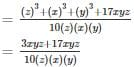= 2

Quantitative Aptitude - Test 9 - Question 5

If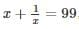find the value of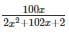Detailed Solution for Quantitative Aptitude - Test 9 - Question 5

Given,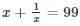⇒ x2 + 1 = 99x
Now,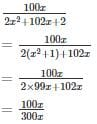= 1/3

Quantitative Aptitude - Test 9 - Question 6

A bicycle wheel makes 5000 revolutions in moving 11 km. The diameter of the wheel, in cm, is

Detailed Solution for Quantitative Aptitude - Test 9 - Question 6

Let the diameter of wheel be D cm.
We know that,
⇒ Distance travelled in 1 revolution × Total no. of revolutions = Total distance travelled
Also, Distance travelled in 1 revolution = Circumference of the wheel
⇒ Circumference of wheel = π × D, where D is the diameter of the wheel
Given, Total number of revolutions = 5000
∴ π × D × 5000 = Total distance travelled
⇒ π × D × 5000 = 1100000 cm (∵ 1 km = 100000 cm)
⇒ D = 70 cm
Hence, the diameter of the wheel is 70 cm.

Quantitative Aptitude - Test 9 - Question 7

If 40 men or 60 women or 80 children can do a piece of work in 6 months, then 10 men, 10 women and 10 children together do half of the work in

Detailed Solution for Quantitative Aptitude - Test 9 - Question 7

Given, 40 men or 60 women of 80 children can do a piece of work in 6 months.
⇒ 40M = 60W =80C
⇒ 2M = 3W = 4C
According to question,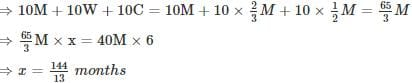So, half of work will be done in number of days = x/2 = 72/13 days
⇒ Half of the work is completed in number of days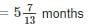Quantitative Aptitude - Test 9 - Question 8

A boat goes 10kms an hour in still water, but takes twice as much time in going the same distance against the current. The speed of the current (in km/hr) is –

Detailed Solution for Quantitative Aptitude - Test 9 - Question 8

Speed of Boat in Current = Speed of Boat in still water – Speed of Current
A boat goes 10 kms an hour in still water
∴ Speed of Boat in still water = 10km/hr
Let it travels for 1 hr and covered 10 km
It takes twice as much time in going the same distance against the current
Thus, it will take 2 hr to cover the same distance of 10 km
∴ Speed of Boat against Current = 10km / 2hrs = 5km/hr
Speed of Current = 10km/hr – 5km/hr = 5km/hr

Quantitative Aptitude - Test 9 - Question 9

A shopkeeper sells a pair of sunglasses at a profit of 25%. If he had bought it at 25% less and sold it for Rs. 20 less, then he would have gained 40%. The cost price of the pair of sunglasses is:

Detailed Solution for Quantitative Aptitude - Test 9 - Question 9

Let the sunglasses were brought for Rs 100.
Selling price be ‘x’ .such that gain% = 25%
Selling price = Rs 100+ Rs 25 = Rs 125
Now if he had bought them at 25% less would mean
⇒ 100 – 25% of 100 = 100 – 25 = Rs 75
Hence let the cost price be Rs 75 and new SP be ‘y’ then
Gain% = 40%
⇒ (SP – CP)/CP × 100 = 40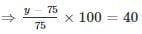⇒ y – 75 = 30
⇒ y = 75 + 30 = 105 Rs
Now if he sells for Previous SP – new SP = Rs. 125 – Rs 105 = Rs 20 less
Then CP = Rs 100
If he sells for Rs 20 less, then C.P.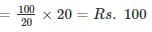Quantitative Aptitude - Test 9 - Question 10

The average age of 8 persons in a committee is increased by 2 years when two men whose ages are 35 years and 45 years are replaced by two new men. The average age of the two new men is (in years)

Detailed Solution for Quantitative Aptitude - Test 9 - Question 10

∵ Average = (Sum total of Ages of All the persons) ÷ (Total no. of persons)
Let the sum of age of 6 persons be x, age of 7th person = 35 & age of 8th Person = 45
⇒ Sum of ages of 8 person = x + 35 + 45 = x + 80
⇒ Average = A = (x + 80)/8
⇒ 8A = x + 80 .... (i)
Let the 7th & 8th person with ages 35 & 45 be replaced with persons having ages as "a" & "b" years respectively
∴ Avg of age of the 2 new men = (a + b)/2
According to question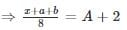⇒ x + a + b = 8A +16... (ii)
∴ From Eq. (i) &Eq (ii) we get
⇒ x + a + b = x + 80 + 16
⇒ a + b = 96
Dividing both sides by 2
⇒ (a + b)/2 = 48

Quantitative Aptitude - Test 9 - Question 11

Two numbers are less than the third number by 20% and 28% respectively. By what per cent is the second number less than the first number?

Detailed Solution for Quantitative Aptitude - Test 9 - Question 11

Let the third number be 100
Then the frist number = 100 – 20 = 80
Second number = 100 – 28 = 72
% with which second number is less than first = (first-second)/first × 100%
⇒ (80 - 72)/80 × 100 %
⇒ 800/80% = 10%

Quantitative Aptitude - Test 9 - Question 12

In an examination, a student gets 20% of total marks and fails by 30 marks. Another student gets 32% of total marks which is more than the minimum pass marks by 42 marks. The pass percentage is

Detailed Solution for Quantitative Aptitude - Test 9 - Question 12

Let the total marks be X
Then, marks obtained by student 1 = 0.2X
Since, student 1 fails by 30 marks after getting 20% marks
∴ According to student1, Passing marks = 0.2X + 30
Similarly, Marks obtained by student 2 = 0.32X
And according to student 2, passing marks = 0.32X - 42;
Equating eq. 1 and 2, we get
0.2X + 30 = 0.32X - 42
⇒ X = 600
∴ Passing marks = (0.2 × 600) + 30 = 150
Passing marks %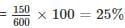Hence, the passing marks are 25%.

Quantitative Aptitude - Test 9 - Question 13

There is 100% increase to an amount in 8 years, at simple interest. Find the compound interest of Rs. 8000 after 2 years at the same rate of interest.

Detailed Solution for Quantitative Aptitude - Test 9 - Question 13

Formulas to be used: -
SI = ( P × r × t ) / 100
For CI: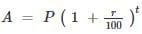Where SI is Simple interest,
A is the amount at the end of time t,
P is the principal,
t is time,
r is rate
For SI, there is 100% increase to amount, thus A = 2P
⇒ SI = p
Time is 8 years.
∴ p = (p × r × t)/100
⇒ r = 100/8 = 12.5%
Now, P = 8000, t = 2years and r = 12.5%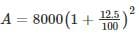⇒ A = 8000 × 1.1252
⇒ A = Rs. 10125
CI = A – P
⇒ CI = 10125 – 8000 = Rs. 2125

Quantitative Aptitude - Test 9 - Question 14

Three students A, B and C play cricket. The runs scored by A and B respectively are in the ratio 3 : 2. B’s runs to C’s runs are also in the same ratio. Together they score 342 runs. Then the runs scored by B are

Detailed Solution for Quantitative Aptitude - Test 9 - Question 14

Given, runs scored by A and B respectively are in the ratio 3 : 2.
∴ A’s score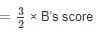Also B’s runs to C’s runs are also in the same ratio.
∴ B’s score : C’s score = 3 : 2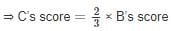Together they scored 342 runs.
∴ A’s score + B’s score + C’s score = 342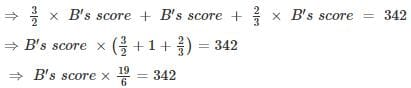⇒ B’s score = 108

Quantitative Aptitude - Test 9 - Question 15

Direction: The following graph shows the production of wheat flour (in 1000 tonnes) by three companies X, Y and Z over the years. Study the graph and answer the questions.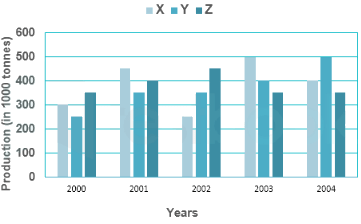Q. The average production for five years was maximum for which company(s)?

Detailed Solution for Quantitative Aptitude - Test 9 - Question 15

From the above chart, we can observe that
Total production of company X during 2000 – 2004 = (300 + 450 +250 + 500 + 400) × 1000 tonnes
= 1900 × 1000 tonnes
Average production of company X during 2000 – 2004 =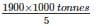= 380 × 1000 tonnes
Total production of company Y during 2000 – 2004 = (250 + 350 + 350 + 400 + 500) × 1000 tonnes
= 1850 × 1000 tonnes
Average production of company Y during 2000 – 2004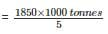= 370 × 1000 tonnes
Total production of company Z during 2000 – 2004 = (350 + 400 + 450 + 350 + 350) × 1000 tonnes
= 1900 × 1000 tonnes
Average production of company Y during 2000 – 2004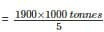= 380 × 1000 tonnes
Hence we can observe that the average production for five years was maximum for X and Z

Quantitative Aptitude - Test 9 - Question 16

Direction: The following graph shows the production of wheat flour (in 1000 tonnes) by three companies X, Y and Z over the years. Study the graph and answer the questions.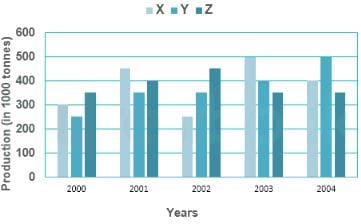Q. What is the percentage increase in the production of company Y from 2002 to 2003?

Detailed Solution for Quantitative Aptitude - Test 9 - Question 16

From the above chart, we can observe thats
Production of company Y in 2002 = 350 × 1000 tonnes
Production of company Y in 2003 = 400 × 1000 tonnes
Percentage increase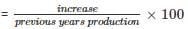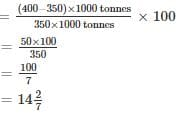Quantitative Aptitude - Test 9 - Question 17

Direction: The following graph shows the production of wheat flour (in 1000 tonnes) by three companies X, Y and Z over the years. Study the graph and answer the questions.Q. What is the ratio of the average production of company X in the period 2002-2004 to the average production of company Y in the same period?

Detailed Solution for Quantitative Aptitude - Test 9 - Question 17

From the above chart, we can observe that
Total production of company X during 2002 – 2004 = (250 + 500 + 400) × 1000 tonnes
= 1150 × 1000 tonnes
Average production of company X during 2002 – 2004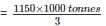Total production of company Y during 2002 – 2004 = (350 + 400 + 500) × 1000 tonnes
= 1250 × 1000 tonnes
Average production of company Y during 2002 – 2004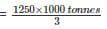Required ratio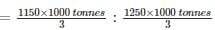= 23 : 25

Quantitative Aptitude - Test 9 - Question 18

Direction: The following graph shows the production of wheat flour (in 1000 tonnes) by three companies X, Y and Z over the years. Study the graph and answer the questions.Q. What is the difference between the production of company Z in 2004 and company Y in 2000 (in thousand tonnes)?

Detailed Solution for Quantitative Aptitude - Test 9 - Question 18

From the above chart, we can observe that
Production of company Z in 2004 = 350 × 1000 tonnes
Production of company Y in 2000 = 250 × 1000 tonnes
Difference = (350 – 250) × 1000 tonnes
= 100 × 1000 tonnes

Quantitative Aptitude - Test 9 - Question 19

If a/b = c/d= e/f = 3, then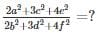Detailed Solution for Quantitative Aptitude - Test 9 - Question 19

Given,
⇒ a/b = c/d= e/f = 3, thus
⇒ a = 3b
⇒ c = 3d
⇒ e = 3f
Putting these values in the given expression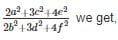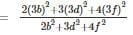Taking out 32 common we get the given expression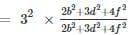= 9
Hence the value of given expression is 9.

Quantitative Aptitude - Test 9 - Question 20

For what value of x is (x + 3264 × 3266) a perfect square,

Detailed Solution for Quantitative Aptitude - Test 9 - Question 20

Given, (x + 3264 × 3266)
= (x + (3265 – 1) × (3265 + 1))
= (x + 32652 – 1)
For this to be a perfect square, x = 1

Quantitative Aptitude - Test 9 - Question 21

Which one of the following three digit numbers divides 9238 and 7091 with the same remainder in each case?

Detailed Solution for Quantitative Aptitude - Test 9 - Question 21

Let the same remainder on dividing these numbers be R.
Hence, (9238 – R) & (7091 - R) both will be divisible by the required three digit number say X.
We know that if X is a factor of (9238 – R) & (7091 - R) then it will also be factor of the difference of (9238 – R) & (7091 - R) i.e.
⇒ X must also be a factor of (9238 – R – (7091 - R)) = 2147
Thus, from given 3 digit numbers only 113 completely divides 2147 and hence its factor.
Hence 113 is the required number that divides 9238 and 7091 to leave same remainder.

Quantitative Aptitude - Test 9 - Question 22

The list price of a digital electronic watch is Rs. 1000. A customer gets two successive discounts on the list price, the 1st being of 15%. Calculate the 2nd discount, if the customer pays Rs. 697 for it.

Detailed Solution for Quantitative Aptitude - Test 9 - Question 22

Given, Marked price = Rs. 1000
After allowing 15%,
Selling price = 1000 – 0.15 × 1000 = 0.85 × 1000 = 850
Let, A successive discount of x% is allowed,
Selling price becomes = 850 – (x/100) × 850
According to the question,
850 – (x/100) × 850 = 697
⇒ 850 (1 – x/100) = 697
⇒ 1 – x/100 = 41/50
⇒ 1 – 41/50 = x/100
⇒ 9/50 = x/100
⇒ x = 900/50 = 18%

Quantitative Aptitude - Test 9 - Question 23

At an instant, the length of the shadow of a pole is √3 times the height of the pole. The angle of elevation of the Sun at that moment is

Detailed Solution for Quantitative Aptitude - Test 9 - Question 23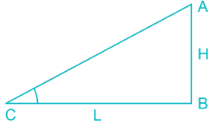From the diagram,
Height of the pole = AB = H
Length of the shadow = BC = L
Given, Length of the shadow is √3 times the height of the pole
∴ BC = L = H√3
From ΔABC
tan ∠ACB = Height/Base
⇒ tan ∠ACB = AB/BC
⇒ tan ∠ACB = H/H√3
⇒ tan ∠ACB = 1/√3
∵ tan 30° = 1/√3
∴ ∠ACB = 30°
∴ Angle of elevation of sun is 30°

Quantitative Aptitude - Test 9 - Question 24

If for some angle θ,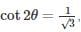then the value of sin 3θ, where 2θ ≤ 90° is

Detailed Solution for Quantitative Aptitude - Test 9 - Question 24

We know that
2θ = cot-1(1/√3) = π/3 for 2θ ≤ 90°
⇒ θ = π/6
⇒ 3θ = π/2
Now, sin 3θ = 1

Quantitative Aptitude - Test 9 - Question 25

If secθ + tanθ = 3, then the value of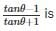Detailed Solution for Quantitative Aptitude - Test 9 - Question 25

(a – b)2 = a2 + b2 – 2ab
Given that, secθ + tanθ = 3
⇒ secθ = 3 – tanθ
Squaring both side,
⇒ sec2θ = 9 – 6×tan θ + tan2θ
We know that, sec2θ = 1 + tan2θ
∴ 1 + tan2θ = 9 – 6 × tanθ + tan2θ
⇒ 6 × tanθ = 8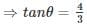Now, we have to calculate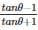Putting the value of tan θ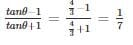## SSC CGL Tier 1 Mock Test Series

42 docs|103 tests
 Use Code STAYHOME200 and get INR 200 additional OFF Use Coupon Code
Information about Quantitative Aptitude - Test 9 Page
In this test you can find the Exam questions for Quantitative Aptitude - Test 9 solved & explained in the simplest way possible. Besides giving Questions and answers for Quantitative Aptitude - Test 9, EduRev gives you an ample number of Online tests for practice

## SSC CGL Tier 1 Mock Test Series

42 docs|103 tests